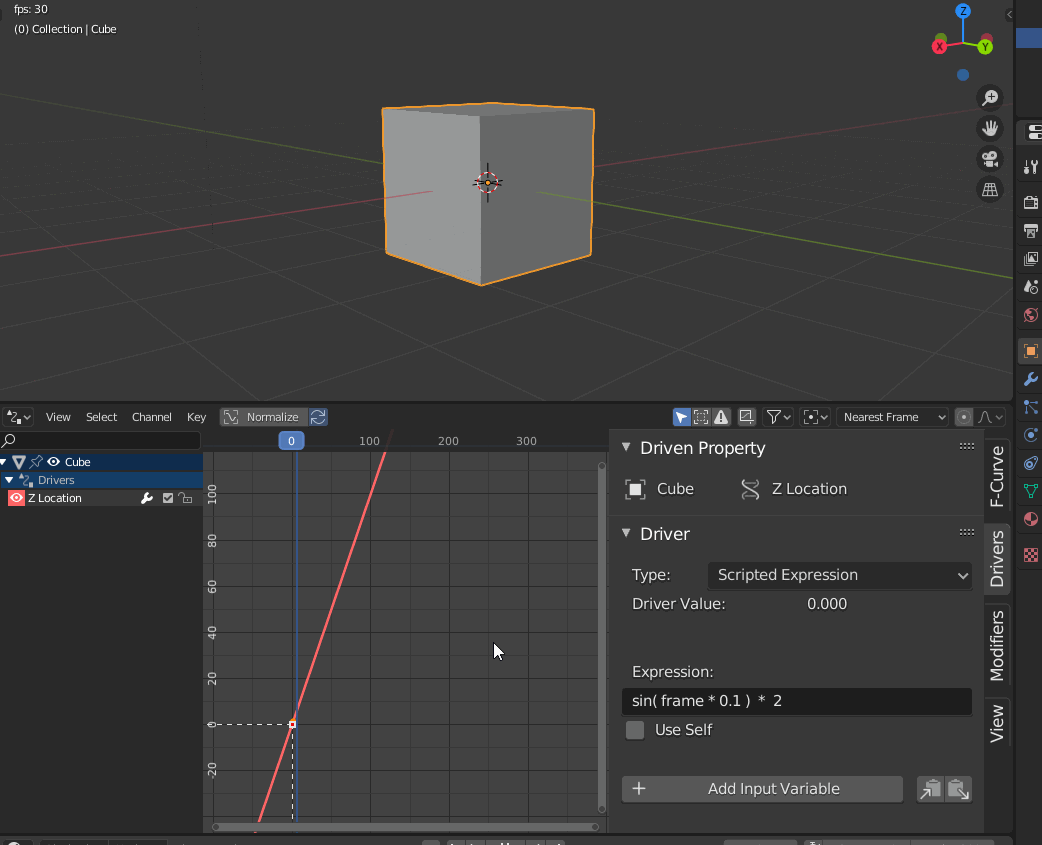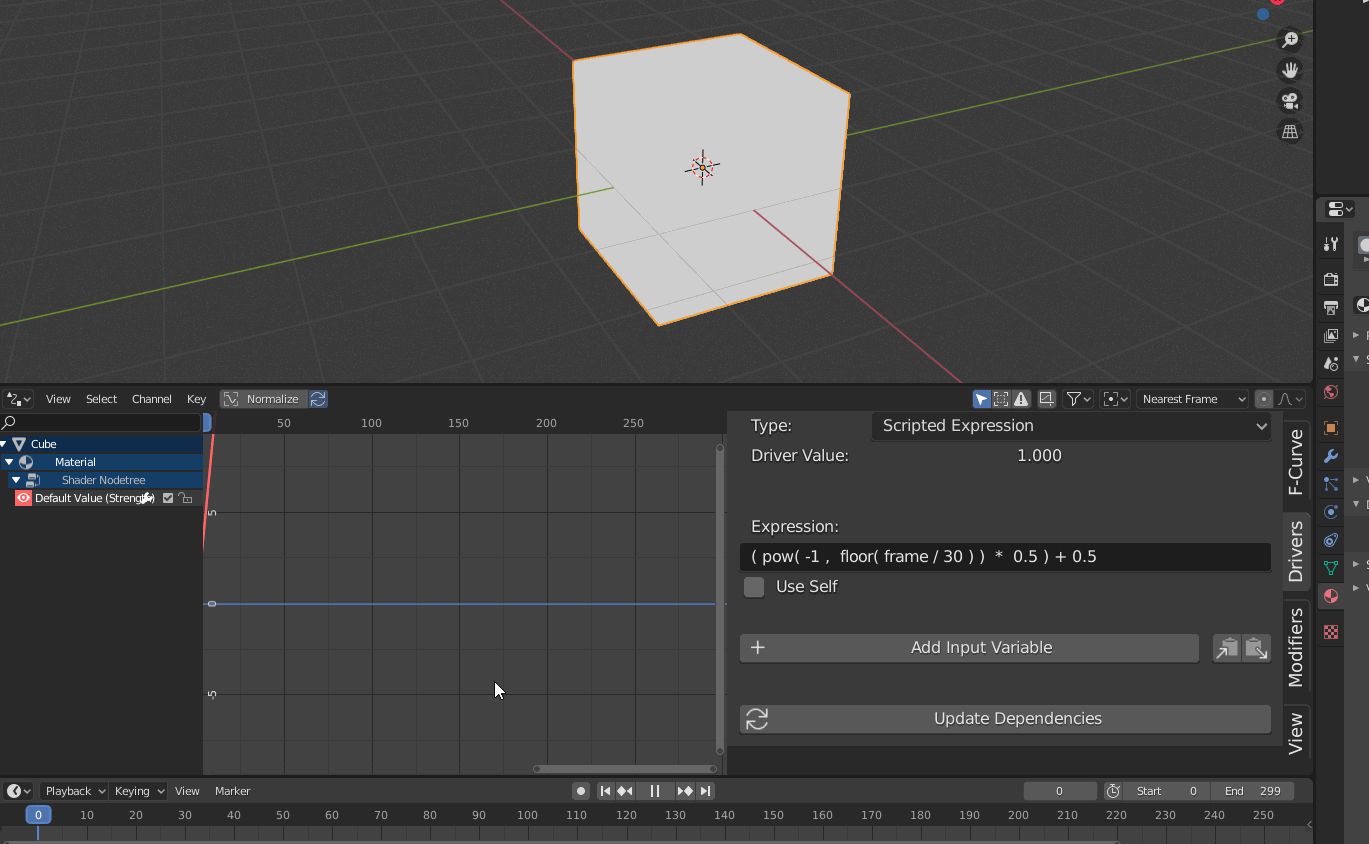## UE4 – Procedural 3D noise bump setups

Software:
Unreal Engine 4.25

Yet another case where I develop my own costly solution only to find out afterwards that there’s actually a much more efficient built-in solution.. 😀

In this case the subject is deriving a bump normal from a procedural or non-uv projected height map/texture (like noise, or tri-planar mapping for example).

The built-in way:
Using the pre-made material functions, PreparePerturbNormalHQ and PerturbNormalHQ, the first of which uses the low level Direct3D functions DDX and DDY to derive the two extra surface adjacent values needed to derive a bump normal, and the last uses the 3 values to generate a world-space bump normal:

1. Noise coordinates are obtained by multiplying the surface shading point local position by a value to set the pattern density.
2. The Noise output value is multiplied by a factor to set the resulting bump intensity.
3. The PreparePerturbNormalHQ function is used to derive the 2 extra values needed to derive a bump normal.
4. The PerturbNormalHQ function is used to derive the World-Space bump normal.
5. Note:
Using this method, the material’s normal input must be set to world-space by unchecking Tangent Space Normal in the material properties.

The method I’m using:
This method is significantly more expensive in the number of shader instructions, but in my opinion, generates a better quality bump.
Sampling 3 Noise nodes at 3 adjacent locations in tangent-space to derive the 3 input values necessary for the NormalFromFunction material function:

1. Noise coordinates are obtained by multiplying the surface shading point local position by a value to set the pattern density.
2. Crossing the vertex normal with the vertex tangent vectors to derive the bitangent (sometimes called “binormal”).
3. Multiplying the vertex-tangent and bitangent vectors by a bump-offset* factor to create the increment steps to the additional sampled Noise values.
* This factor should be parameter for easy tuning, since it determines the distance between the height samples in tangent space.
4. The increment vectors are added to the local-position to get the final height samples positions.
5. The NormalFromFunction material function is used to derive a tangent-space normal from the 3 supplied height samples.

Note:
From my experience, even though the UV1, UV2 and UV3 inputs of the NormalFromFunction are annotated as V3, the function will only work is the inputs are a scalar value and not a vector/color.

## 3ds max – Using a GradientRamp procedural texture in Mapped mode

Software:
3ds max 2020

Using the GradientRamp procedural texture map in Mapped mode can very useful for creating procedural material effects.
The Idea is that the lightness value from a different map will determine what part of the GradientRamp is sampled.

In this example the GradientRamp uses values produced by a procedural Falloff map set to Perpendicular-Parallel mode, as its coordinates source, to create richly colored metal that changes its Hue depending on View/Incident angle:In this example the GradientRamp uses values produced by a procedural Noise map as its coordinates source to create an irregular color effect:Note:
The examples here were rendered using V-Ray Next for 3ds max, but this technique could also be used with other rendering engines.

Related:

## Blender – Basic time-dependent animation driver examples

Software:
Blender 2.82

To setup a time-dependent Driver in Blender, simply use the built-in frame variable.
In this example the expression:

`sin(frame)`

Set as a Z axis location driver for the cube causes it to oscillate up and down:Changing the expression to:

`sin( frame * 0.1 ) * 2`

Causes the motion to be twice as high and 10X slower:In this example, the expression:

`( pow( -1 ,  floor( frame / 30 ) )  *  0.5 ) + 0.5`

Set to the cube’s Emission shader’s Strength attribute causes it to alternate between values of 0 and 1 every second (30 frames in this case):## Python for 3ds max – Animated Mesh

Software:
3ds max 2019

This is an example of procedurally animating a mesh’s vertices via Python script.Notes:
1. The model has to be converted to Editable Mesh before the script is run.
* unless the scrip will be extended to do it.
2. The model must be selected for the script to work.

```import MaxPlus
import math
from MaxPlus import INode
from MaxPlus import TriObject
from MaxPlus import SelectionManager
from MaxPlus import Factory
from MaxPlus import Animation
from MaxPlus import Point3
from MaxPlus import Control

ticks_frame = 160

#Selection
sel = []
for n in SelectionManager.Nodes:
sel.append(n)
node = sel

#Setup Controllers
obj = node.GetObject()
Tri = TriObject._CastFrom(obj)
mesh = Tri.GetMesh()
num_verts = mesh.GetNumVertices()
mesh_anim = obj.GetSubAnim(0)
pnt_ctrl = Factory.CreateDefaultMasterPointController()
node.AssignController(pnt_ctrl,1)
for i in range(num_verts):
bezp3 = Factory.CreateDefaultPoint3Controller()
bezp3.SetPoint3Value(mesh.GetVertex(i))
mesh_anim.AssignController(bezp3,i)

#Animation
Animation.SetAnimateButtonState(True)
for t in range(100):
time = t * ticks_frame
Animation.SetTime(time)